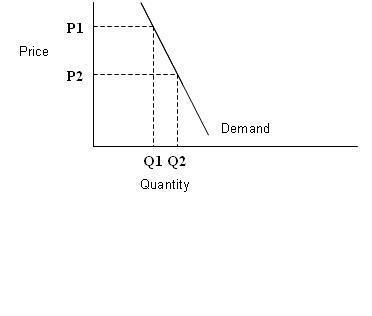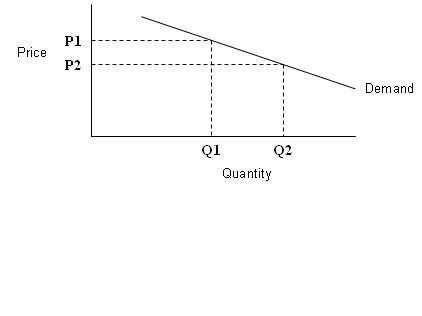# Elastic And Inelastic Demand

181

ELASTIC AND INELASTIC DEMAND

Slope and elasticity of demand have an inverse relationship. When slope is high elasticity of demand is low and vice versa.

When the slope of a demand curve is infinity, elasticity is zero (perfectly inelastic demand); and when the slope of a demand curve is zero, elasticity is infinite (perfectly elastic demand). Unit elasticity means that a 1% change in price will result in an exact 1% change in quantity demanded. Thus elasticity will be equal to one. A unit elastic demand curve plots as a rectangular hyperbola. Note that a straight line demand curve cannot have unit elasticity as the value of elasticity changes along the straight line demand curve. Virtual University

Inelastic demand curve PictureElastic demand curve Picture